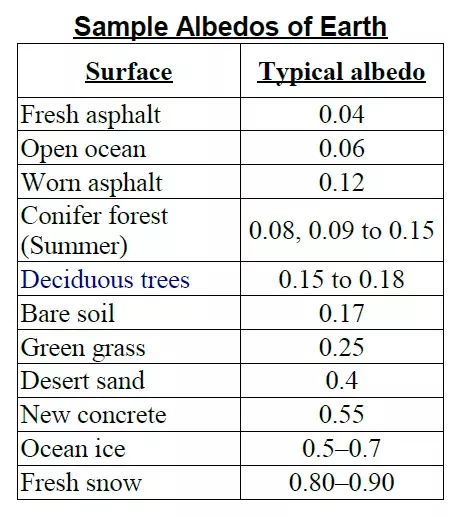## Basics of Photo Voltaic

In this tutorial we are going to build some basic solar systems. First we will have a look at the V-I characteristic of a solar panel and from there we will use the solar panel together with a MPP controller for feeding into an AC grid. Finally we will look at solar powered battery charging.

The required components can be found in the Components/Library and the figures below show where to find them.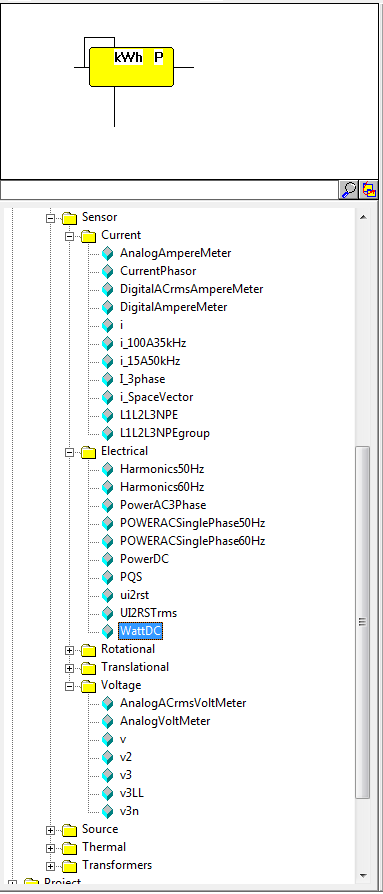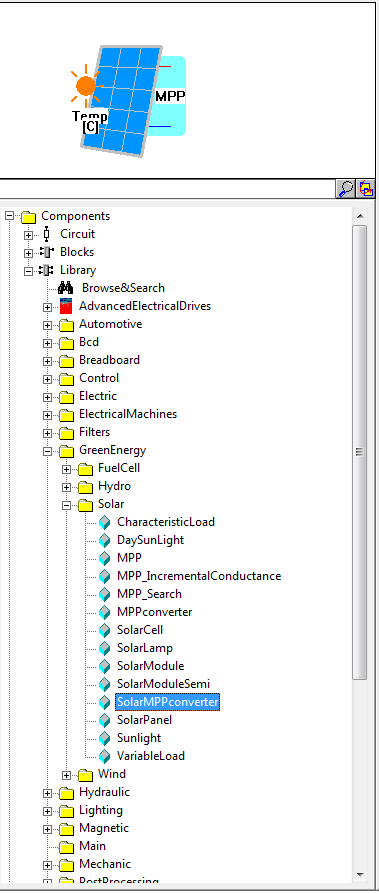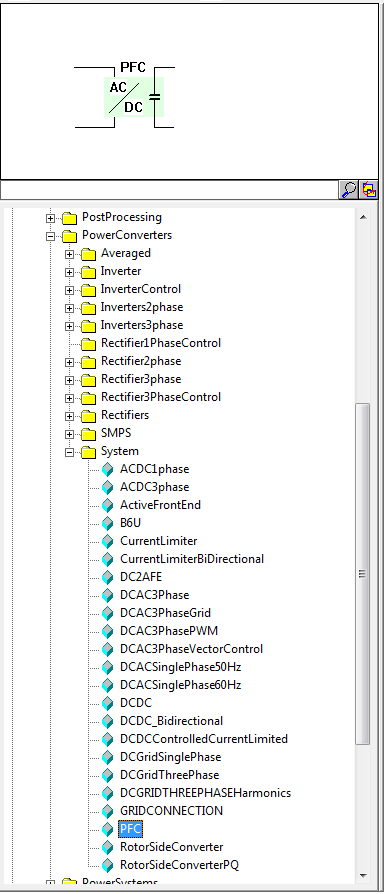nog een keer */?>## Solar panel V-I characteristics

By using the characteristic-load component from the greenenergy\solar library, a complete V-I characteristic can be created. This is useful if the characteristic is not known on beforehand, since it draws the characteristic including $$V_{oc}$$ and $$I_{oc}$$. Change the solar insulation in the sunlight block and rerunning the simulation shows the variation of the maximum current on the sunlight intensity. Changing the internal series and parallel resistance will show the dependence of the characteristic directly.

Note!
Take care to specify (at least) 1 second of simulation time (Tscreen=1) to get the full overview of the characteristic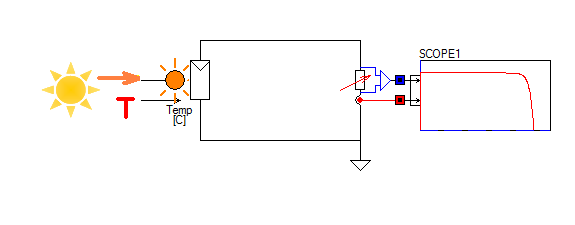Figure 1: Basic characteristic of a solar panel.

### Component parameters

Components / Circuit:

SOLARCELL1[greenenergy\solar\solarcell.lib](Isc=5, Rseries[ohm]=10m, Rparallel=100k, Uoc[Volt]=35, Tnominal=25, alphaI[A/Celcius]=2.06m, alphaU[V/Celcius]=-0.152)
GROUND1[electric\ground\ground.lib]

Tscreen = 1
Integration stepsize (Euler) = 1m

## Solar delivering to the AC single phase grid

Delivering solar energy to the main grid requires two power electronic components, A Maximum Power Point [MPP] tracker and a grid connected inverter. The model for the MPP is included in the solar panel model. Here the power for Normal Operating Cell Temperature [NOTC] can be supplied. The connection to the single phase grid is done via the component PFC, being a single phase grid inverter with power factor equal to 1.

The single phase grid converter PFC is connected to a single phase grid and can have bidirectional power flow, depending on the DC side voltage. The voltage level at the DC side of the PFC is a parameter inside the PFC component and in the example below it is set to 500V. The PFC component regulates the DC voltage to this pre-set value. Current flowing from the solar panel into the DC link will increase the DC link voltage. The PFC is now regulating towards its pre-defined value by delivering current into the AC grid. At startup, when the DC link voltage has to be established first, power flows from the single phase AC grid into the DC link.

The MPP component is delivering the electric energy into the DC link. It requires a constant DC link voltage to operate, which is supplied and regulated by the PFC component. The MPP controller converts the optimum solar panel voltage level up to the DC link voltage. Depending on the solar intensity, the maximum current the solar panel is delivering is changing. The maximum power point is where maximum power is generated by the solar panel for that specific solar intensity and temperature. The MPP controller converters the voltage and current levels such that $V_{MPP} \cdot I_{MPP} = V_{DC} \cdot I_{DC}$ as the power balance has to stay intact. As a result of this the amount of current flowing into the DC link always equals: $I_{DC} = \frac{V_{MPP} \cdot I_{MPP} }{V_{DC}}$ where the voltage $$V_{DC}$$on the DC link is constant and $$V_{MPP}$$ and $$I_{MPP}$$ vary with the sunlight intensity and temperature.

The current flowing into the AC grid equals $V_{DC} \cdot I_{DC} = PF \cdot V_{AC} \cdot I_{AC}$ where $$PF$$ is the power factor at the AC side. Since the power factor is maintained equal to $$1$$ by the PFC component, the current flowing into the AC grid equals $I_{AC} = \frac{ V_{DC} \cdot I_{DC} }{V_{AC}}$ where the the term $$V_{DC} \cdot I_{DC}$$ can be replaced by $$V_{MPP} \cdot I_{MPP}$$ such that $I_{AC} = \frac{ V_{MPP} \cdot I_{MPP} }{V_{AC}}$ Since the AC voltage level is nearly constant, it becomes clear that the amplitude of the AC current $$I_{AC}$$ is linearly depending on the power generated by the solar panel $I_{AC} \equiv P_{MPP}$

### What is the difference between STC and NOCT in photovoltaic module specifications?

Different voltage, current and power ratings are reported for STC (Standard Test Conditions) and NOCT (Normal Operating Cell Temperature). What is the difference between both sets of technical specs?

Standard Test Conditions are the laboratory conditions under which all PV modules are tested. It can be said that STC is a benchmark for comparing different types of PV modules, even if they are not from the same provider.

STC:

• An irradiance of 1000 watts per square meter, which simulates peak sunshine on a surface directly facing the sun in a day without clouds.
• A surface temperature of 25°C
• A light spectrum that closely simulates sunlight: AM 1.5 G

However, these are idealized conditions which don't reflect the real site conditions under which a PV module will operate. The conditions at Nominal Operating Cell Temperature  [NOTC]aim to simulate reality more closely.

NOTC:

• The irradiance is 800 watts per square meter, which takes into account the fact that PV modules don't always face the sun. It also considers atmospheric or geographic conditions what might diminish sunshine.
• Solar panels heat up considerably during operation, so the temperature considered is 45 (+/- 3) °C.
• The light spectrum is the same as for STC.
• A windspeed of 1 m/s is considered, with air at 20°C

This means that solar panels will always have higher ratings at STC compared with NOTC.

The amount of power delivered to the AC grid is measured by the two blocks POWERDC and POWERACSINGLEPHASE50HZ from Components/Library/sensor/electrical an displayed in the the top scope [Average AC and DC power]. The block POWERACSINGLEPHASE50HZ averages the amount of power over a period of $$20ms = \frac{1}{50Hz}$$. The instantaneous amount of power delivered to the single phase AC grid is displayed in the bottom scope4. Here you can clearly see that the amount of power equals $\hat{I}_{AC}sin(2 \pi f t) \cdot \hat{V}_{AC}sin(2 \pi f t) = \hat{P}_{AC}sin^2(2 \pi f t)$ Every $$20ms$$ the amount of power delivered is nearly zero. No wonder a three-phase connection is used in higher power applications.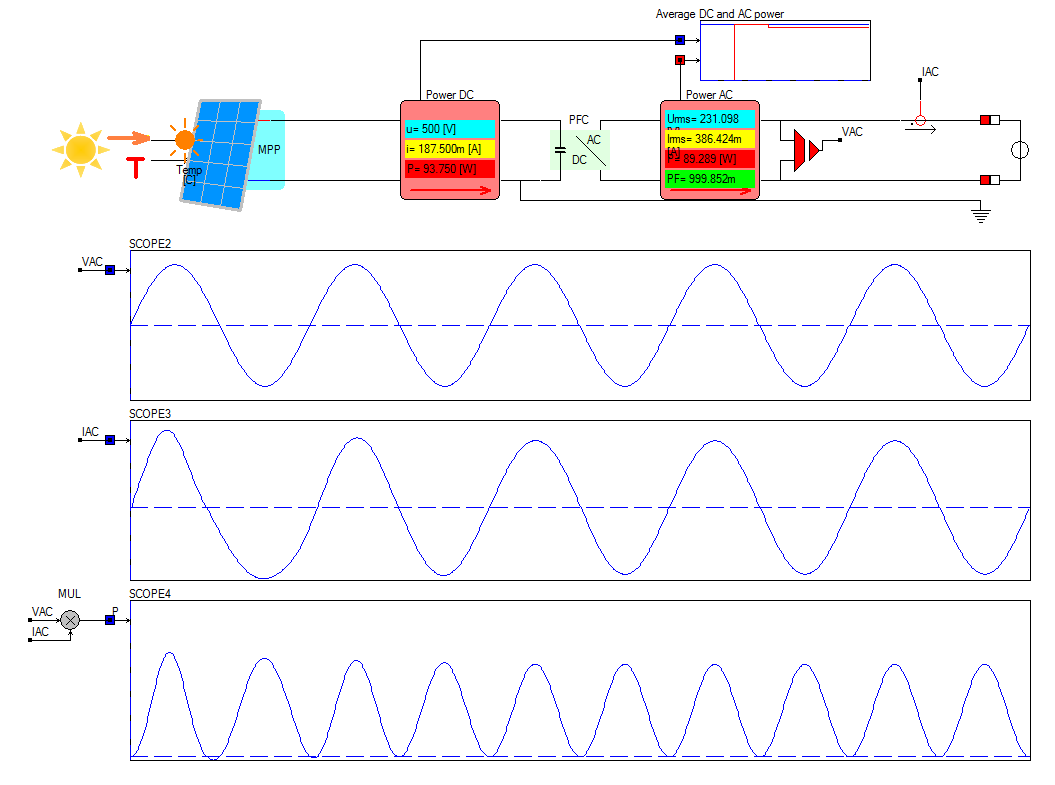Figure 2: Solar panel with MPP and PFC connected to a single phase grid.

### Component Parameters

Components / Circuit:

Components / Block:P[math/mul]
Components / Library:GRID1[powersystems\grid\singlephase\grid.lib](Xneutral=100m, Rneutral=160m, Rphase=240m, Xphase=150m, Frequency(Hz)=50, Phase(0-360)=0, VLLrms=400)
I1[sensor\current\i.lib](R=1.5m)
V21[sensor\voltage\v2.lib](Gain=1)
POWERACSINGLEPHASE50HZ1[sensor\electrical\poweracsinglephase50hz.lib](F[Hz]=50)
PFC1[powerconverters\system\pfc.lib](PowerFactor[0..1]=1, Efficiency[0..100]=95, Vdc[V]=500, InitialACVoltage[V]=230, Q[VAR]=0)
POWERDC1[sensor\electrical\powerdc.lib]
SOLARMPPCONVERTER1[greenenergy\solar\solarmppconverter.lib](TC_mpp=-0.2, NOCT[C]=45, Pmpp[Watt]=100)

Tscreen = 100m
Integration stepsize = 100u

## Solar charging of a battery

In this tutorial we can see how the solar panel is performing during a single day. The amount of sunlight is modeled using the component daysunlight. Its output is the solar intensity from 0:00 until 24:00, where the maximum solar intensity is specified by the parameter SunlightDensity[Watt/m2].

The generated solar power is charging a Lead Acid battery. The stored amount of energy is measured by the WattDC block, which monitors the delivered kilowatts per hour [kWh]. Scope 1 and scope 2 show the solar intensity and generated current, while scope 3 and scope 4 show the State of Charge [SoC] and energy [kWh].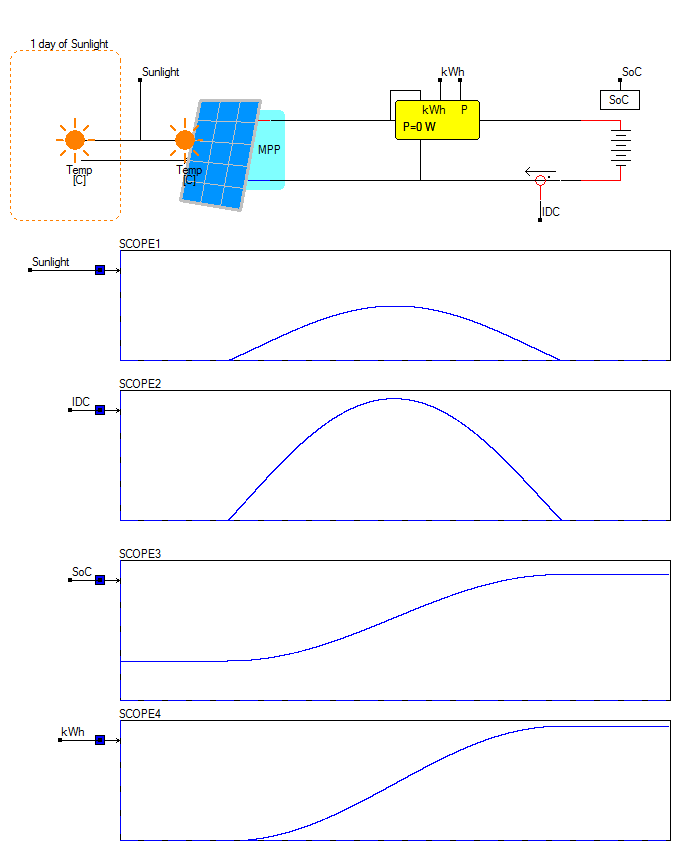Figure 3: Solar charging a battery.

### Component Parameters

Components / Circuit:Components / Block:Components / Library:SOLARMPPCONVERTER1[greenenergy\solar\solarmppconverter.lib](Pmpp[Watt]=100, NOCT[C]=45, TC_mpp=-0.2)
DAYSUNLIGHT1[greenenergy\solar\daysunlight.lib](Temperature[Celsius]=25, SunlightDensity[Watt/m2]=1000)
WATTDC1[sensor\electrical\wattdc.lib]
I1[sensor\current\i.lib](R=1.5m)
BATTERY1[electric\battery\battery.lib](U[volt]=200, inital_SoC[0..100]=20, Rinternal[ohm]=10m, kWh=2)

Tscreen = 86.400k
Integration stepsize = 5

### Some notes on the blockdiagram Semiconductor/Semi:solar P1=5 model

Parameter:
• P1=5
Inputs:
1. Time
Central European Time in seconds, Connect to the block Time
2. Not-used
3. Panel tilt angle
The optimum tilt angle is calculated by subtracting 15°to your latitude during winter, and adding 15° from your latitude during summer. For example, in Europe a tilt angle of 35 degrees optimizes winter irradiation.
4. Panel orientation
Solar panels are most effective when installed on south-facing roofs. Facing East is equal to -90°, facing West equals an inputvalue of +90°
5. Latitude
Latitude ranges from 0° at the Equator to 90° at the North-pole and -90° at the South-pole. Amsterdam=52°, Berlin=52°, Rio=-42°
6. Longitude
Specifies east-west. Greenwich=0°, Amsterdam=4°, Berlin=13.4°, Rio=-22°.
7. Albedo coefficient
A default value of 0.2 is used for normal circumstances
8. Damping
Weather conditions, 1=ideal(no weather conditions), 0=average weather conditions

To simulate a single day, set Tscreen=24*60*60=86400.

To simulate a complete year, set Tscreen=365*24*60*60=365*86400=31536000.Albedo is another name for reflectivity. The albedo of a surface determines how much sunlight will be absorbed and warm the surface compared to another surface that reflects most of the light and does not change temperature.